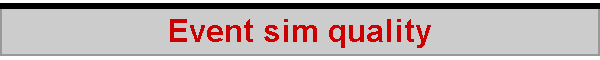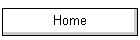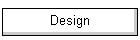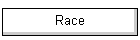With help from Anders Wallin, I took a closer look at the quality of the random numbers generated by QBASIC in the simulation.  In an "average" run, the simulator might track 50 boats, generating 7 random numbers each time, through 20 races per event, and 50 events per run.  Add other numbers required from time to time, and this is about half a million numbers.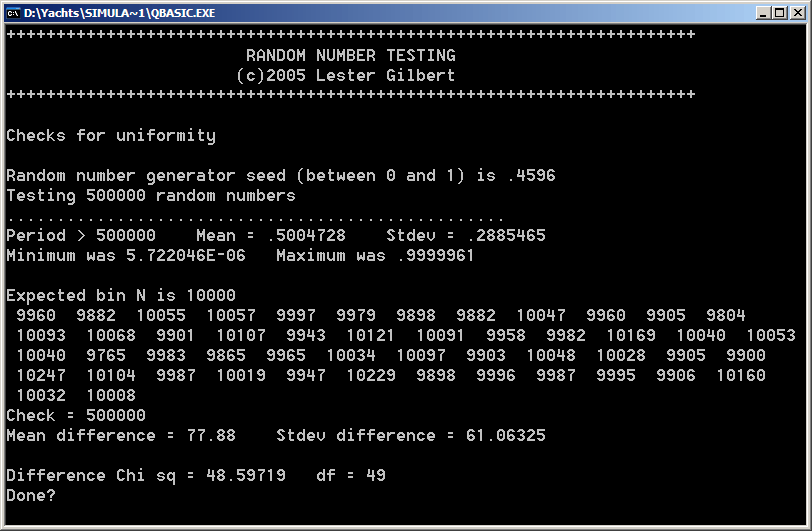Basic quality indicators The first issue to check was that that the RND generator had a period larger than 500,000.  Turns out it did, so this means that no numbers were repeated, every one was unique.  The second issue was to check that these numbers were more or less evenly spread in the interval [0 to 1[, and the graph showed acceptable spread.  (The interval was divided into 50 'bins', and a count was made for each random number that fell into the relevant bin.)  The Chi squared measure of the deviation from perfection was 48.6, and this was excellent (df=49, p approx 0.50).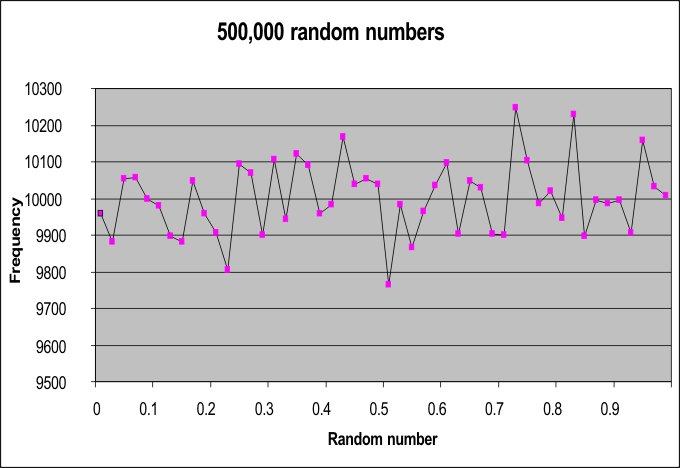Detailed chi-sq indicator Detailed analysis of the uniformity of the random number stream was carried out by examining the numbers in batches of 1,000.  For each batch, their smoothness in the [0..1[ interval was measured by chi squared.  Given 500 batches, there were 500 resulting chi-sq values, and their distribution was analysed for their variability.  As before, the [0..1[ interval was divided into 50 bins, so the resulting chi squared distribution had df=49.  From tables of chi squared, it was possible to determine the expected number of batches which would have average smoothness and how many would have excessive smoothness - either too rough or too smooth.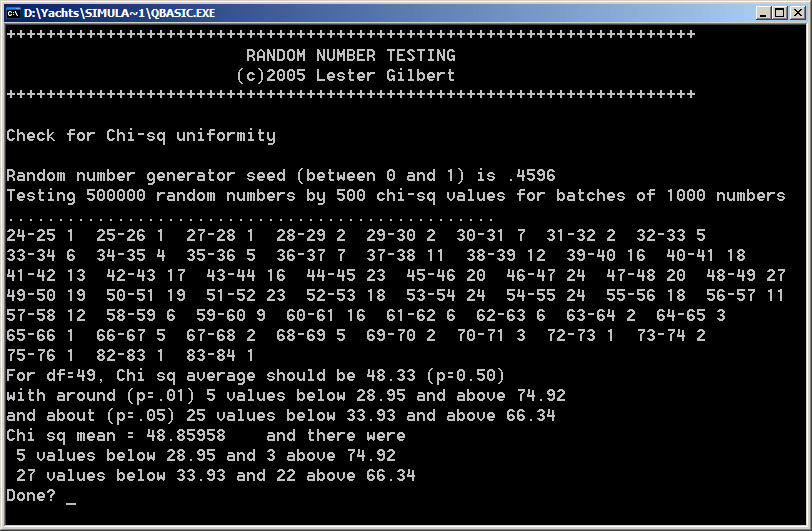The graph shows the distribution of the chi squared values, and this again was very good.  For example, theory said that about 25 chi-sq values should be below 33.93, and it turned out that there were 27 batches with chi-sq below this value.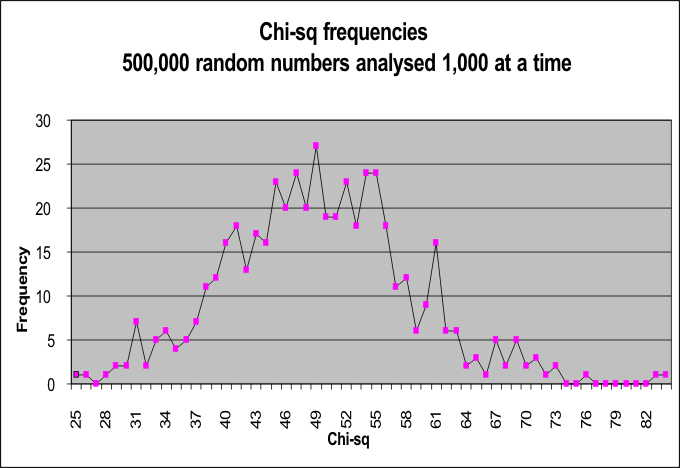Autocorrelation It was a long time since I've analysed random distributions, and I was looking forward to seeing how the QBASIC RND function fared on this pretty tough test.  The idea here is that, given one random number X(i), the immediately following number X(i+1) should not have any similarity.  This is measured by the autocorrelation.  Autocorrelations were calculated for successive numbers separated by 1, 2, 3, .. up to 14 steps.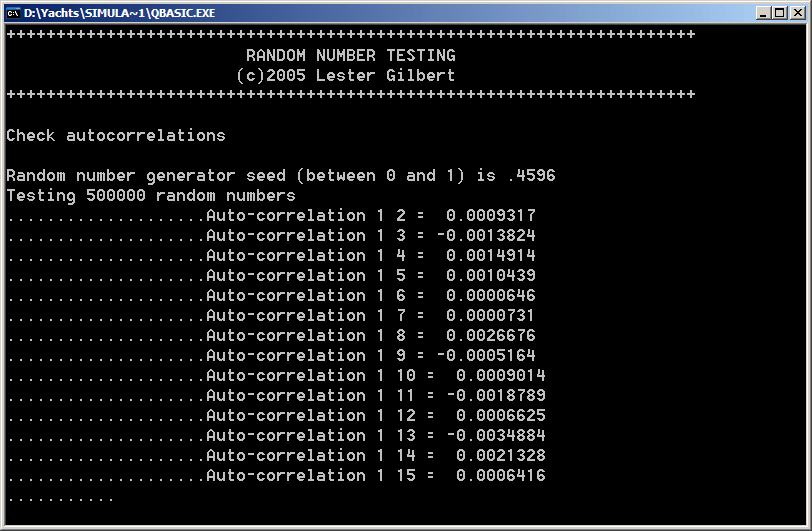The graph shows good, but not outstanding, autocorrelation properties.  They are good, in that there is quite negligible correlation between one random number X(i) and a closely-following number X(i+k).  Even the highest correlation coefficient observed, r=-.0035, is close enough to 0 to be fine.  More problematic is the fact that there is a distinct pattern for even k, and for odd k.  Even k autocorrelations are almost all negative, and odd k autocorrelations are almost all positive.  The pattern isn't large, however.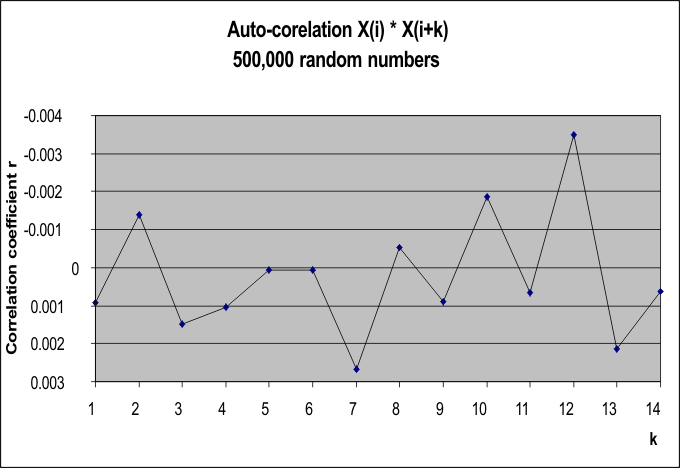I thought I'd explore the quality of the autocorrelations further.  The following graph illustrates the results of measuring the k=1 and k=2 autocorrelations for 1000 random numbers at a time.  The other k=3 and so on autocorrelations show similar kinds of results.  There is (random) deviation from the expected distribution, and two of the computed chi-squared goodness-of-fit statistics are significant (at the p=0.05 level) -- which is one more than would be expected anyway given the run was up to k=19.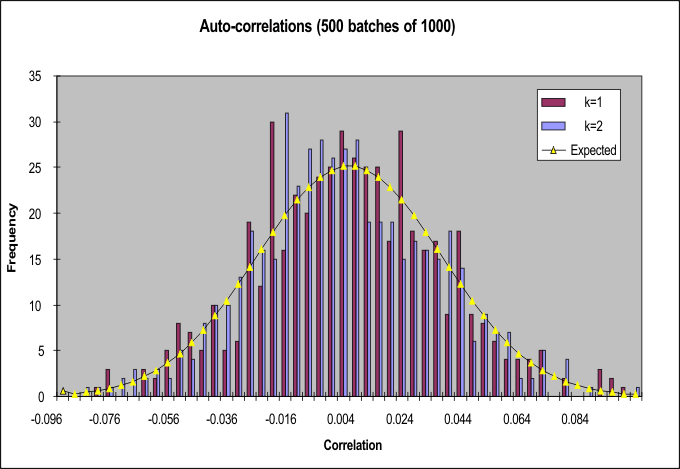```k Chi p 1 65.0 0.06 2 39.4 0.84 3 51.7 0.37 4 48.6 0.49 5 76.0 0.01 6 41.6 0.76 7 48.9 0.48 8 57.6 0.19 9 70.6 0.02 10 57.9 0.18 11 50.5 0.42 12 60.0 0.13 13 51.2 0.39 14 56.5 0.22 15 38.5 0.86 16 44.1 0.67 17 40.0 0.82 18 54.6 0.27 19 57.3 0.19```  I finally ran a quick check of the quality of the pseudo-Gaussian distribution, to see how well the rather crude method of simply averaging 6 random numbers would work.  The graph appears to show, visually, that it works rather well.  In detail, however, there is a highly significant discrepancy between the expected normal curve and the actual results (chi-squared = 211, df = 49, p << .01).  There are too many pseudo-normal numbers in the 0.5 to 1.5 standard deviation region, and too few around the mean and in the tails.  If you look really carefully, you might see this on the graph...  But this is not that important a failing in this simulation, which does not require excellence in the Gaussian properties of its numbers.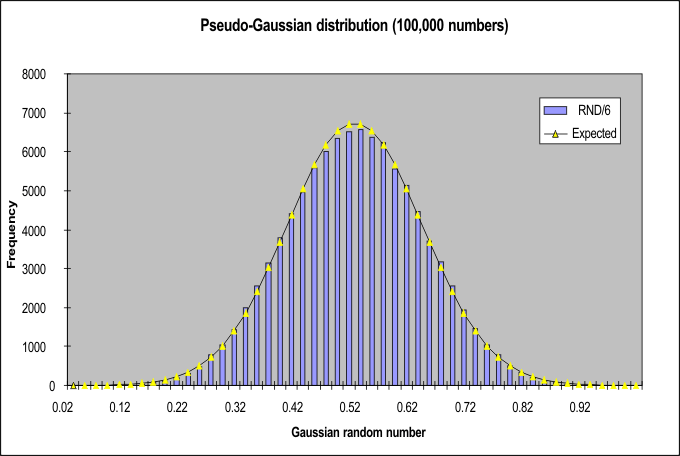So, the QBASIC RND function is not nearly as bad as I feared!